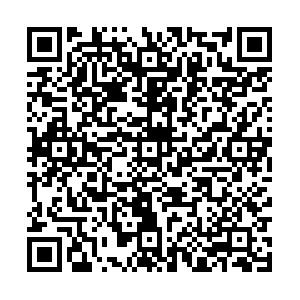## 留言板引用本文: 杨钧超, 陈向明, 邹鹏, 等. 复合材料层合板剪切稳定性试验及强度预测[J]. 复合材料学报, 2022, 40(0): 1-11Junchao YANG, Xiangming CHEN, Peng ZOU, Zhe WANG. Shear stability test and strength prediction of composite laminates[J]. Acta Materiae Compositae Sinica.
 Citation: Junchao YANG, Xiangming CHEN, Peng ZOU, Zhe WANG. Shear stability test and strength prediction of composite laminates[J]. Acta Materiae Compositae Sinica.## 复合材料层合板剪切稳定性试验及强度预测

###### 通讯作者: 陈向明，博士，高级工程师，研究方向为复合材料结构强度　E-mail: asrichenxm@avic.com
• 中图分类号: V257；TB330.1

## Shear stability test and strength prediction of composite laminates

Funds: National Key R&D Program of China (2019 YFA0706800); National Natural Science Foundation of China(52005458); Aviation Science Foundation of China (2020 Z055023002)
• 摘要: 对无损伤及含冲击损伤的复合材料层合板进行了剪切稳定性试验，基于数字图像相关方法(Digital image correlation，DIC)对层合板屈曲后屈曲行为进行了实时测量。试验结果表明：引入冲击损伤后，复合材料层合板剪切屈曲波形、屈曲载荷无明显变化，失效模式转变，承载能力下降了9.69%。随后，基于断裂面失效理论，建立了考虑剪切非线性效应的复合材料渐进损伤失效模型，并对复合材料层合板剪切失效过程进行了模拟。模型采用软化夹杂法将冲击损伤等效简化，直接将损伤区的几何边界信息写入材料模型中，不需要对冲击损伤区进行切割，从而保证了整体网格质量。与试验结果对比发现：模型考虑剪切非线性对屈曲载荷预测无明显影响，对后屈曲承载能力的预测精度影响较大，不考虑剪切非线性效应时的误差可达20%以上；软化夹杂法可以有效地模拟冲击损伤，预测的含冲击损伤的复合材料层合板的屈曲载荷、破坏载荷误差分别为−3.17%、−1.27%。

• 图  1  CCF300/BA3202复合材料层合板尺寸

Figure  1.  Dimensions of CCF300/BA3202 composite laminates

图  2  T4试件冲击支持方式及分层尺寸

Figure  2.  Impact support mode and delamination size of T4 specimen

图  3  无损伤试件(T1~T3)应变片分布及编号

Figure  3.  Strain gauges distributions and numbers of undamaged specimens(T1~T3)

图  4  CCF300/BA3202复合材料层合板剪切试验方法

Figure  4.  Shear test method for CCF300/BA3202 composite laminates

图  5  基体潜在断裂面上的应力分量

Figure  5.  Stress components on potential fracture surface of matrix

${\sigma _1}$, ${\sigma _2}$, ${\sigma _3}$, ${\tau _{12}}$, ${\tau _{13}}$, ${\tau _{23}}$, ${\tau _{21}}$, ${\tau _{31}}$, ${\tau _{32}}$—Stress components in the material's principal axis coordinate system; ${\theta _{{\text{fp}}}}$—Angle of the potential fracture surface of matrix; ${\sigma _{\text{n}}},\;{\tau _{{\text{nt}}}},\;\;{\tau _{{\text{nl}}}}$—Stress components on potential fracture surface of matrix

图  6  CCF300/BA3202复合材料的剪切非线性行为

Figure  6.  Shear nonlinear behavior of CCF300/BA3202

图  7  CCF300/BA3202复材层板剪切试验有限元模型

Figure  7.  Finite element model for shear test of CCF300/BA3202 composite laminates

MPC—Multi-point constraints

图  8  T4试件等效冲击损伤区域

Figure  8.  Equivalent impact damage area of T4 specimen

F4—Impact damage field variable

图  9  T1试件载荷-应变曲线

Figure  9.  Load-strain curve of T1 specimen

图  10  无损伤的T1试件屈曲及失效时的面外位移云图

Figure  10.  Out-of-plane displacement cloud diagram of undamaged T1 specimen during buckling and failure

W—Out of plane displacement

图  11  含冲击损伤的T4试件屈曲及失效时的面外位移云图

Figure  11.  Out-of-plane displacement cloud diagram of T4 specimen with impact damage during buckling and failure

图  12  几何缺陷因子和剪切非线性对CCF300/BA3202复材层板屈曲载荷破坏载荷的影响

Figure  12.  Effects of geometric defect factor and shear nonlinearity on buckling load and failure load of CCF300/BA3202 composite laminates

图  13  刚度折减系数对CCF300/BA3202复材层板破坏载荷的影响

Figure  13.  Effects of stiffness reduction factor on failure load of CCF300/BA3202 composite laminates

图  14  T4试件仿真的屈曲模态

Figure  14.  Simulated buckling mode of T4 specimen

U3—Out of plane displacement

图  15  T4试件仿真的失效模式

Figure  15.  Simulated failure mode of T4 specimen

F1—Fiber damage field variable; F2—Matrix damage field variable

图  16  T4试件预测的载荷-位移曲线

Figure  16.  Predicted load-displacement curve of T4 specimen

图  17  T4试件纤维间损伤扩展过程

Figure  17.  Inter-fiber failure propagation process of T4 specimen

表  1  CCF300/BA3202碳纤维增强环氧树脂复合材料性能参数

Table  1.   Material properties of CCF300/BA3202 carbon fiber reinforced epoxy composite

 E1/GPa E2/GPa G12/GPa ν12 118 8.98 4.21 0.306 XT/MPa XC/MPa YT/MPa YC/MPa 1835 1296 82.5 240 SL/MPa GIC/(N·mm−1) GIIC/(N·mm−1) 166 0.744 1.90 Notes：${E_1}$—Longitudinal elastic modulus; ${E_2}$—Ransverse elastic modulus; ${G_{12}}$—Shear modulus ${\nu _{{\text{12}}}}$—Poisson's ratio; ${X_{\text{T}}}$—Longitudinal tensile strength; ${X_{\text{C}}}$—Longitudinal compressive strength; ${Y_{\text{T}}}$—Transverse tensile strength; ${Y_{\text{C}}}$—Transverse compressive strength; ${S_{\text{L}}}$—Shear strength; GIC—Mode I fracture toughness ; GIIC—Mode II fracture toughness.

表  2  材料性能退化方案

Table  2.   Material performance degradation scheme

 Fail mode No damage zone Impact damage zone None - $\begin{gathered} {E_1} \to \eta {E_1} \hfill \\ {E_2} \to \eta {E_2} \hfill \\ G_{12}^{{\text{eq}}} \to \eta G_{12}^{{\text{eq}}} \hfill \\ \end{gathered}$ Fiber failure $\begin{gathered} {E_1} \to (1 - {d_{{\text{FF}}}}){E_1} \hfill \\ {E_2} \to (1 - {d_{{\text{FF}}}}){E_2} \hfill \\ G_{12}^{{\text{eq}}} \to (1 - {d_{{\text{FF}}}})G_{12}^{{\text{eq}}} \hfill \\ {\nu _{12}} \to (1 - {d_{{\text{FF}}}}){\nu _{12}} \hfill \\ \end{gathered}$ $\begin{gathered} {E_1} \to (1 - {d_{{\text{FF}}}})\eta {E_1} \hfill \\ {E_2} \to (1 - {d_{{\text{FF}}}})\eta {E_2} \hfill \\ G_{12}^{{\text{eq}}} \to (1 - {d_{{\text{FF}}}})\eta G_{12}^{{\text{eq}}} \hfill \\ {\nu _{12}} \to (1 - {d_{{\text{FF}}}}){\nu _{12}} \hfill \\ \end{gathered}$ Inter-fiberfailure $\begin{gathered} {E_2} \to (1 - {d_{{\text{IFF}}}}){E_2} \hfill \\ G_{12}^{{\text{eq}}} \to (1 - {d_{{\text{IFF}}}})G_{12}^{{\text{eq}}} \hfill \\ {\nu _{12}} \to (1 - {d_{{\text{IFF}}}}){\nu _{12}} \hfill \\ \end{gathered}$ $\begin{gathered} {E_2} \to (1 - {d_{{\text{IFF}}}})\eta {E_2} \hfill \\ G_{12}^{{\text{eq}}} \to (1 - {d_{{\text{IFF}}}})\eta G_{12}^{{\text{eq}}} \hfill \\ {\nu _{12}} \to (1 - {d_{{\text{IFF}}}}){\nu _{12}} \hfill \\ \end{gathered}$ Notes：${E_1}$—Longitudinal elastic modulus; ${E_2}$—Transverse elastic modulus; $G_{12}^{{\text{eq}}}$—Equivalent shear modulus; ${\nu _{{\text{12}}}}$—Poisson's ratio; η—Stiffness reduction factor; ${d_{{\text{FF}}}}$—Fiber damage state variable; ${d_{{\text{IFF}}}}$—Matrix damage state variable.

表  3  CCF300/BA3202复材层板剪切试验结果

Table  3.   Shear test results of CCF300/BA3202 composite laminates

 Number Buckling load/kN Fracture load/kN Test Average Test Average T1 64.6 64.2 153.5 156.8 T2 65.0 156.2 T3 63.1 160.7 T4 63.0 141.6
•点击查看大图
##### 计量
• 文章访问数:  54
• HTML全文浏览量:  37
• 被引次数: 0
##### 出版历程
• 收稿日期:  2022-02-28
• 录用日期:  2022-05-20
• 修回日期:  2022-05-10
• 网络出版日期:  2022-06-09

### 目录/下载:  全尺寸图片 幻灯片
• 分享
• 用微信扫码二维码

分享至好友和朋友圈